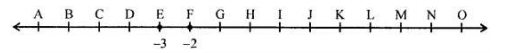# ML Aggarwal Solutions Class 7 Mathematics Solutions for Integers Exercise 1 in Chapter 1 - Integers

A number line representing integers is given below:The integers -3 and -2 are marked by points E and F respectively. Which integers are marked by points B, D, H, J, M, and O?

Integers which are marked by points B, D, H, J, M and O are, B = -6 D = -4 H = 0 J = 2 M = 5 O = 7

Related Questions
Exercises

Lido

Courses

Teachers

Book a Demo with us

Syllabus

Maths
CBSE
Maths
ICSE
Science
CBSE

Science
ICSE
English
CBSE
English
ICSE
Coding

Terms & Policies

Selina Question Bank

Maths
Physics
Biology

Allied Question Bank

Chemistry
Connect with us on social media!